# Mathematics at Aksharam

Want to share an opinion?—drop a mail

Dr. Lalitha Subramanian, About the Author

# CBSE X Mathematics

## CHAPTER 1: Real Numbers

### 1-1: Euclid's Division Lemma

Lemma: Given positive integers $a$ and $b$, there exist unique integers $q$ and $r$ such that $a = bq + 4$, $0 \leq r \lt b$.
When we divide an integer $a$ by another integer $b$, we get a quotient $q$ and a remainder $r$. The remainder $r$ is always positive and less than the divisor $b$. This lemma is further illustrated in the following example:

#### Example 1:

Illustrate Euclid's Lemma for each of the following pairs of integers: $(a)$ $42 \div 5$; $(b)$ $75 \div 4$

Solution:

$(a)$ When we divide $42$ by $5$, we get quotient $8$ and remainder $2$. So, Euclid's Lemma shows that $42 = 5 \times 8 + 2$. Note that the remainder $r =2 \lt 5$.
$(b)$ When we divide $75$ by $4$, we get remainder $18$ and remainder $3$. The Lemma shows that $75 = 4 \times 18 + 3$. Here again, note that $r = 3 \lt 4$.

Euclid Lemma gives us a good technique to find the HCF of two positive integers.The following example illustrates how the algorithm works:

#### Example 2:

Find the HCF of each of the following pairs of positive integers: $(a)$ $560, 38$; $(b)$ $4052, 420$

Solution:

Divide the bigger number by the smaller number and find the quotient. If the remainder is not $0$, then divide the divisor by the remainder and continue this process until you get a remainder $0$. The divisor of the last division is the required HCD. The processis shown below:
$(a)$ \begin{eqnarray} 560 &=& 38 \times 14 + 28\\ 38 &=& 28 \times 1 + 10\\ 28 &=& 10 \times 2 + 8\\ 10 &=& 8 \times 1 + 2\\ 8 &=& 2 \times 4 + 0\\ \end{eqnarray}
The divisor of the last step is $2$. So, HCF of $560$ and $38$ is $2$.
$(b)$ \begin{eqnarray} 4052 &=& 420 \times 9 + 272\\ 420 &=& 272 \times 1 + 148\\ 272 &=& 148 \times 1 + 124\\ 148 &=& 124 \times 1 + 24\\ 124 &=& 24 \times 5 + 4\\ 24 &=& 4 \times 6 + 0\\ \end{eqnarray}
The HCF of $4052$ and $420$ is $4$, as that is the divisor of the last step.

Apart from finding HCF of two large numbers, Euclid's algorithm is very useful in computer programmins, and also several applications, this algorithm can be extended to all integers except $0$. One such application is related to properties numbers. The following example shows one such application.

#### Example 3:

Show that any positive integer of the form $2m-1$, where $m$ is any integer, is odd.

Solution:

Let $a$ be any positive integer of the form $2m-1$ where $m$ is any integer. If $a$ is not odd, then it should be even. This means that $a = 2q$ for some positive integer $q$. This results in $2m-1 = 2q$. Rearranging the terms, we get $2m-2q = 1$. Factoring $2$ and rewriting, we get $2(m-q) = 1$. Now, as $m$ and $q$ both are integers, $m-q$ is also an integer. Let $m-q=b$ for some integer $b$. This gives us $2b = 1$, so that $b = \frac{1}{2}$. This contradicts the truth that $b$ is some integer. So, our assumption that $a$ is even is false.Thus, we show thatany positive integer of the form $2m-1$ is always odd.

The example below shows use of the algorithm for find HCF of large numbers in word problems:

#### Example 4:

A book seller has $384$ fiction books and $216$ non-fiction books. He wants to stack these books in such a way that each stack has the same number, the take up the least area in his shelf. What is the least number of books that can be placed in each stack?

Solution:

Here, the HCF of $384$ and $216$ gives the maximum number of books that can be stacked in order to consume least space. \begin{eqnarray} 384 &=& 216 \times 1 + 168\\ 216 &=& 168 \times 1 + 48\\ 168 &=& 48 \times 3 + 5\\ 48 &=& 24 \times 2 + 0\\ \end{eqnarray} So, stacking each type of books such that 24 books are in a row would consume least space.

## Practice Problems

For problems # 1 - 5, use Euclid's algorithm to find the HCF:

$260 = 125 \times 2 + 10; 125 = 10 \times 12 + 5; 10 = 5 \times 2 + 0$; So, HCF = $5$

$875 = 255 \times 3 + 90; 255 = 90 \times 2 + 75; 90 = 75 \times 1 + 15; 75 = 15 \times 5 + 0$. So, HCF = $15$

$12620 = 624 \times 20 + 140; 624 = 140 \times 4 + 64; 140 = 64 \times 2 + 12; 64 = 12 \times 5 + 4; 12 = 4 \times 3 + 0$. So, HCF= $4$.

$570 = 315 \times 1 + 255; 315 = 255 \times 1 + 60; 255 = 60 \times 4 + 15; 60 = 15 \times 4 + 0$. So, HCF = $15$

$2636 = 296 \times 8 + 68; 296 = 68 \times 4 + 24; 68 = 24 \times 2 + 20; 24 = 20 \times 1 + 4; 20 = 4 \times 5 + 0$. So, HCF = $4$.

Proof similar to example $3$.

Proof similar to example $3$.

Maximum number of $8$ columns.

Maximum length of each piece is $6$ inches

He should pack his order in $11$ boxes, with $30$ breads in each box.

$9$ liter bottles; $24$ botltles of the first kind and $31$ bottles of the second kind.

$28$ and $49$

$108$ and $84$

She can prepare $4$ plates, with each plate containing $3$ chicken pieces and $4$ vegetale rolls.

### 1-2: Fundamental Theorem of Arithmetic

Definition: Every composite number can be expressed as a product (as factors) of prime numbers in a unique way, apart from the order in they are written.

#### Example 1:

Write the prime factorization of each of the following numbers: $(a)$ $600$; $(b)$ $4851$; $(c)$ $7875$

Solution:

$(a)$ From the tree diagram shown below, we get $600 = 2^3 \times 3 \times 5^2$. Though these factors can be written in any order, they will have three $2$'s, one $3$, and two $5$'s.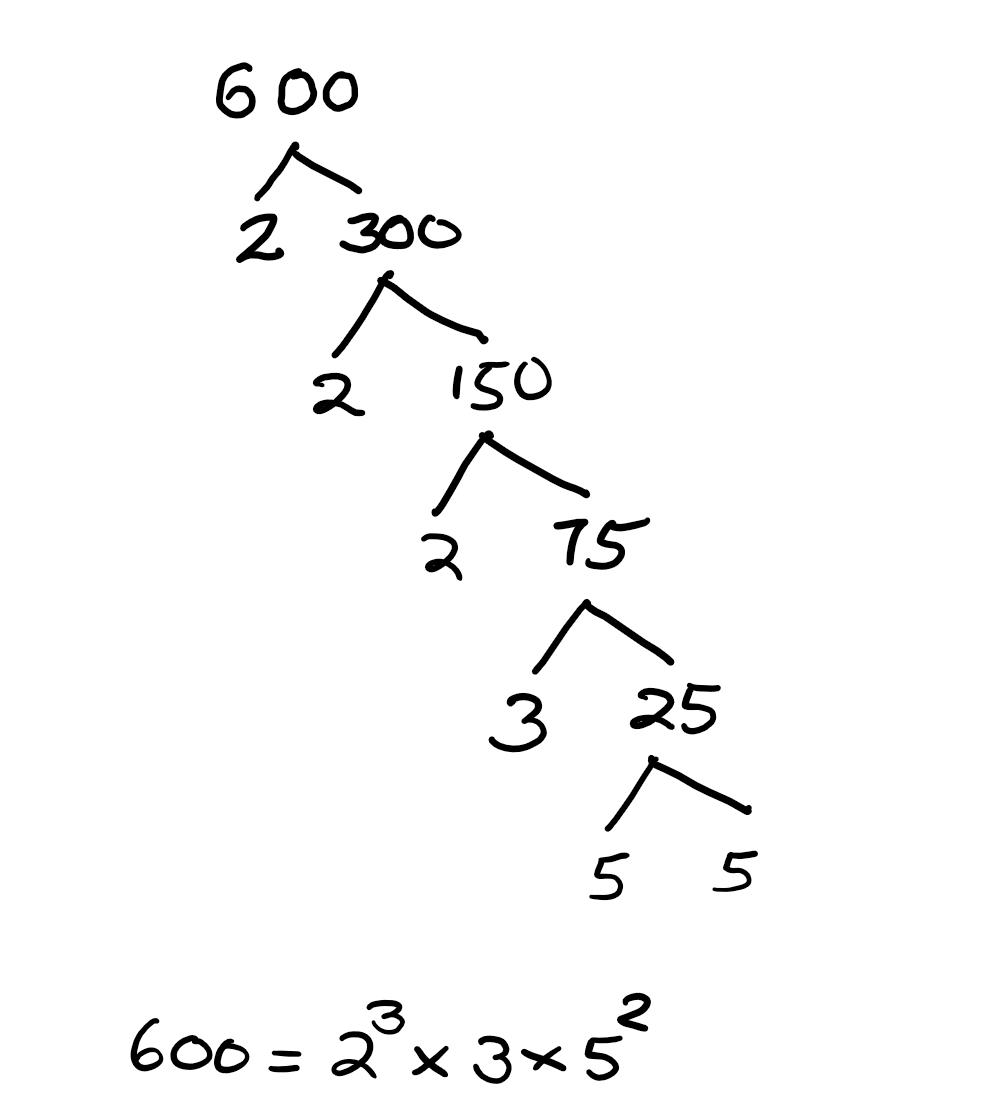$(b)$ From the tree diagram, we get $4851 = 3^2 \times 7^2 \times 11$, which is unique except for the order in which these factors can be written.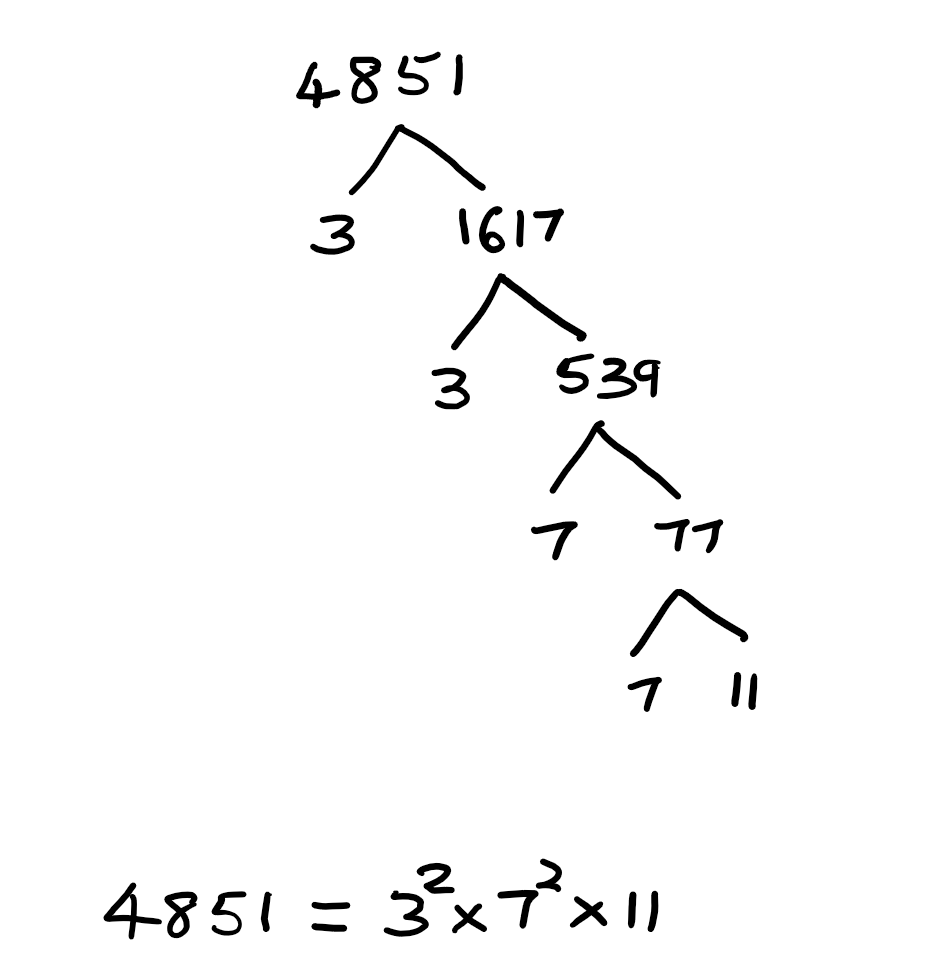$(c)$ From the tree diagram, we get, $7875 = 3^2 \times 5^2 \times 7$.The Fundamental Theorem of Arithmetic has many applications. The examples illustrated below show some applications in number theory.

#### Example 2:

Show that the numbers of the form $3^n$ where $n$ is a natural number cannot end with the digit $2$

Solution:

If possible, let a number $3^n$ for a natural number $n$ end with $2$. This would mean that $2$ is a factor of $3^n$ as it becomes an even number. But, we know that $3^n = 3 \times 3 \times 3......$, where $3$ occurs $n$ times. So, our presumption that $3^n$ ends with $2$ for some $n$ is false. This means that any number of the form $3^n$ for a natural number $n$ cannot end with $2$.

Another important revelation made possible by the Fundamental Theorem of Arithmetic is regarding the HCF and LCM of two positive integers. This is illustrated clearly in the following example.

#### Example 3:

Find the HCF and LCM of $24$ and $30$ by prime factorization method.

Solution:

Prime factorization of $24 = 2 \times 2 \times 2 \times 3 = 2^3 \times 3^1$. Prime factorization of $30 = 2 \times 3 \times \ 5$. HCF = $2 \times 3 = 6$. LCM = $2^3 \times 3 \times 5 = 120$.

From the above example, we note that HCF x LCM = $6 \times 120 = 720$. Product of the two positive integers is $24 \times 30 = 720$. This relationship is true for any pair of positive integers. Thus, the property says:
Given two positive integers $a$ and $b$, HCF ($a, b$) x LCM ($a, b$) = $a \times b$.

Following example illustrate methods to solve problems using this preoperty.

#### Example 4:

Find the HCF of $120$ and$176$ by prime factorization method. Hence find their LCM.

Solution:

Prime factorization of $120 = 2^3 \times 3 \times 5$. Prime factorization of $176 = 2^4 \times 11$. HCS (120, 176) is the product of smallest powers of the prime factors: $2^3 = 8$. Using the property discussed above, we have LCM = $\frac{120 \times 176}{8} = 2640$.

#### Example 5:

HCF and LCM of two positive integers is $9$ and $6930$ respectively. If one of the numbers is $198$, find the other number.

Solution:

Let the other number be $x$. Then, we have \begin{eqnarray} 198x &=& 9 \times 6930\\ x &=& \frac{9 \times 6930}{198}\\ x &=& 315\\ \end{eqnarray}.

Another use of the Fundamental Theorem of Arithmetic is the ease of finding LCM and HCF of more than two positive integers. We use the fact that HCF of two of more positive integers is the product of the smallest powers of all the common prime factors occuring all the numbers and the LCM is the product of the highest powers of all the prime factors occuring in any one or more of the numbers. Following examples uses this fact to find the LCM and HCF of given numbers.

#### Example 6:

Find the HCF and LCM of $18$, $21$, and $33$ by prime factorization method.

Solution:

\begin{eqnarray} 18 &=&2 \times 3^2\\ 21 &=& 3 \times 7 \\ 33 &=& 3 \times 11\\ HCF &=& 3\\ LCM &=& 2 \times 3^2 \times 7 \times 11\\ &=& 1386\\ \end{eqnarray}

#### Example 7:

Find the HCF and LCM of $12$, $45$ and $72$ by prime factorization method.

Solution:

\begin{eqnarray} 12 &=& 2^2 \times 3\\ 45 &=& 3^2 \times 5\\ 72 &=& 2^3 \times 3^2\\ HCF &=& 3\\ LCM &=& 2^3 \times 3^2 \times 5\\ &=& 360\\ \end{eqnarray}

Please remember that product of three positive integers is not equal to the product of their LCM and HCF.

## Practice Problems

For problems # 1 - 5, express each number as a product of prime factors.

$3^2 \times 17$.

$3 \times 5 \times 19$

$2 \times 7 \times 11 \times 13$

$2^5 \times 3 \times 67$

$2 \times 3 \times 13^2$

For problems $6 - 10$, find LCM and HCF using prime factorization.

$306 = 2 \times 3^2 \times 17$ and $675 = 3^3 \times 5^2$. So, HCF = $3^2 = 9$ and LCM = $2 \times 3^3 \times 5^2 \times 17 = 22,950$.

$39 = 3 \times 13$ and $91 = 7 \times 13$. So, HCF = $13$ and LCM = $3 \times 7 \times 13 = 273$ .

$72 = 2^3 \times 3^2$ and $120 = 2^3 \times 3 \times 5$. So, HCF = $2^3 \times 3 = 24$ and LCM = $2^3 \times 3^2 \times 5 = 360$

$6 = 2 \times 3$, $15 = 3 \times 5$, and $42 = 2 \times 3 \times 7$. So, HCF = $3$ and LCM = $2 \times 3 \times 5 \times 7 = 210$

$69 = 3 \times 23$, $92 = 2^2 \times 23$, and $115 = 5 \times 23$. So, HCF = $23$ and LCM = $2^2 \times 3 \times 5 \times 23 = 1380$

$2184$

$2520$

$56, 108$ and $72, 84$.

$765$.

$12$.

### 1-3: Revisiting Irrational Numhers

A number is called an irrational number if it cannot be written in the form of a fraction, i.e, in the form of $\frac{p}{q}$, where $p,q$ are integers, coprime, and $q\neq 0$. Moreover, while rational numbers can be written as terminating or recurring decimals, irrational numbers are written as decimal that are non-terminating and non-recurring. Examples of irrational numbers are $\sqrt{2}$, $\sqrt{3}$, etc. Generally, nth root of any number that is not nth power of a number is irrational. That is, $\sqrt[n]{a}$, where $a$ is not the nth power of any integer. The decimal expansion of $\sqrt{2} = 1.41421356237........$, which is neither terminating, nor recurring.
In order to prove that an irrational number cannot be represented as a fraction, we need a theorem based on the Fundamental Theorem of Arithmetic:
Theorem: If a prime number $p$ divides a number $a^2$ where $a$ is any positive integer, then $p$ divides $a$ also.

This theorem basically indicates that all prime factors of $a$ occurs twice in the prime factorization of $a^2$ and so, if $p$ divides $a^2$, then it is a prime factor of $a^2$. So, it must occur twice in the prime factorization of $a^2$. So, $p$ would be a prime factor of $a$ too.

The examples below illustrate the process of using the above theorem to prove that a number is irrational.

#### Example 1:

Prove that $\sqrt{5}$ is irrational.

Solution:

Let us assume that $\sqrt{5}$ is rational. Then, we should be able to express $\sqrt{5}$ as a fraction $\frac{p}{q}$, where $p,q$ are coprime integers with $q\neq 0$. That is $\sqrt{5} = \frac{p}{q}$. Squaring both sides, we get $5 = \frac{p^2}{q^2}$. Rearranging, we get $p^2 = 5q^2$. This means that $5$ is a factor of $p^2$. Then, by the above theorem, $5$ should also be a factor of $p$. Let $p = 5k$ for some integer $k$. So, $p^2 = (5k)^2 = 25k^2$. Subtituting for $p$, we have $25k^2 = 5q^2$. Diving both sides by $5$ gives $q^2 = 5k^2$. This means that $5$ is a factor of $q$. That is, $5$ is a factor of both $p$ and $q$. This contradicts the fact the $p$ and $q$ are coprime. So, our assumption is false. Hence, $\sqrt{5}$ is an irrational number.

#### Example 2:

Prove that $2 - \sqrt{5}$ is irrational.

Solution:

Let us assume that $2 - \sqrt{5}$ is rational. Then, it can be expressed as $\frac{p}{q}$, where $p,q$ are coprime integers, and $q \neq 0$. That is, $2 - \sqrt{5} = \frac{p}{q}$. Rearranging, we get $\sqrt{5} = 2 - \frac{p}{q} = \frac{2q - p}{q}$ As $p$ and $q$ are integers, this would mean that $\sqrt{5}$ is expressed as a fraction $\frac{2q-p}{q}$, and so $\sqrt{5}$ is rational. But we know that $\sqrt{5}$ is irrational, which is a contradiction. So, our assumption is false. Hence, $2 - \sqrt{5}$ is irrational.

#### Example 3:

Prove that $3 + 4 \sqrt{2}$ is irrational.

Solution:

Let us assume that $3 + 4\sqrt{2}$ is rational. Then $3 + 4\sqrt{2} = \frac{p}{q}$, where $p$ and $q$ are coprime integers and $q \neq 0$. Rearranging this equation, we get $4\sqrt{2} = \frac{p}{q} - 3 = \frac{p - 3q}{q}$. This gives $\sqrt{2} = \frac{p-3q}{4q}$. Since $p$ and $q$ are integers, $p-3q$ and $4q$ are also integers.This means that $\sqrt{2}$ can be expressed as a fraction, which makes it a rational number. This contradicts the fact that $\sqrt{2}$ is irrational. So, our assumption is false. Thus, $3 + 4\sqrt{2}$ is irrational.

#### Example 4:

Prove that $\frac{\sqrt{7}}{2\sqrt{3}}$ is irrational.

Solution:

Let us assume that $\frac{\sqrt{7}}{2\sqrt{3}}$ is rational. Then $\frac{\sqrt{7}}{2\sqrt{3}} = \frac{p}{q}$, where $p$ and $q$ are coprime integers and $q \neq 0$. Rearranging this equation, we get $q\sqrt{7} = 2p\sqrt{3}$. Squaring both sides gives $7q^2 = 12p^2$. So, $q^2 = \left(\frac{12}{7}\right)p^2$. As $p$ and $q$ are coprime integers, by the theorem, $\frac{12}{7}$ is a factor of $q$ also. That is, $q = \left(\frac{12}{7}\right)r$ for some interger $r$. So, we end up with $q^2 = \left(\frac{12}{7}\right)^2r^2 = \left(\frac{12}{7}\right)p^2$. Thus we have $p = \left(\frac{12}{7}\right)r$. This means that $p$ and $q$ are not coprime integers. This is a contradiction. Hence our assumption is falso. So, $\frac{\sqrt{7}}{2\sqrt{3}}$ is irrational.

## Practice Problems

See to examples

See to examples

See to examples

Similar to example 2

See to examples

See to examples

See to examples

See to examples

### 1-4: Revisiting Decimal Expansion of Rational Numbers

We have discussed in earlier classes process of conversion of fractions into decimals and decimals into fractions. A rational number can be expressed as a fraction $\frac{p}{q}$, where $p$ and $q$ are coprime integers, and $q \neq 0$, which has either terminating or repeating decimal expansion.

Following examples illustrate an important property of fractions that have terminating decimal expansions:

#### Example 1:

Find decimal expansions of $(i) \frac{3}{8}; \quad (ii) \frac{12}{5} \quad (iii) \frac{9}{20} \quad (iv) \frac{67}{40}$

Solution:

We know that place values of decimal expansions are tenths, hundredths thousandths, etc. Therefore, if we can write an equivalent fraction for each of the fractions such that the denominators are powers of ten, then we can write their decimal expansions without actual division.
$(i)$ The number $8$ can be made power of ten ($10^3$) by multiplying it by $125$ \begin{eqnarray} \frac{3}{8} &=& \frac{3}{8} \times \frac{125}{125}\\ &=& \frac{3\times 125}{8 \times 125}\\ &=& \frac{375}{1000}\\ &=& 0.375\\ \end{eqnarray}
$(ii)$ The number $5$ multiplied by $2$ gives $10$: \begin{eqnarray} \frac{12}{5} &=& \frac{12 \times 2}{5 \times 2}\\ &=& \frac{24}{10}\\ &=& 2.4\\ \end{eqnarray}
$(iii)$ The number $20$ multiplied by $5$ gives $100$: \begin{eqnarray} \frac{9}{20} &=& \frac{9 \times 5}{20 \times 5}\\ &=& \frac{45}{100}\\ &=& 0.45\\ \end{eqnarray}
$(iv)$ The number $40$ multiplied by $25$ gives $1000$: \begin{eqnarray} \frac{67}{40} &=& \frac{67 \times 25}{40 \times 25}\\ &=& \frac{1675}{1000}\\ &=& 1.675\\ \end{eqnarray}

Since $10 = 5\times 2$, all powers of $10$ have factors of $2$s and $5$s. Consequently, any fraction whose denominators have only $2$s and $5$s as factors can be written as equivalent forms with denominators as powers of ten. This means that these fractions have terminating decimals as their decimal expansion. The following examples illustrate that any terminating decimal, when converted as fraction, has denominators whose factors contain only $2$s and $5$s.

#### Example 2:

Express each of the following decimals as fractions: $(i) 0.325; \quad ii) 2.25; \quad (iii) 3.728$.

Solution:

$(i)$ \begin{eqnarray} 0.325 &=& \frac{325}{1000} \\ &=& \frac{25 \times 13}{25 \times 40}\\ &=& \frac{13}{40}\\ &=& \frac{13}{2^3 \times 5}\\ \end{eqnarray}
$(ii)$ \begin{eqnarray} 2.25 &=& 2\frac{25}{100}\\ &=& 2\frac{1}{4}\\ &=&2 \frac{1}{2^2}\\ \end{eqnarray}
$(iii)$ \begin{eqnarray} 3.726 &=& 3\frac{728}{1000}\\ &=& 3\frac{182}{225}\\ &=& 3 \frac{182}{3^2 \times 5^2}\\ \end{eqnarray}

On the other hand, rational numbers whose fraction forms have denominators with factors other than $2$s and/or $5$s have repeated decimal expansions. Following examples illustrate this property:

#### Example 3:

Express each of the following fractions as decimals: $(i) \frac{2}{3} \quad (ii) \frac{11}{6} \quad (iii) \frac{5}{9} \quad (iv) \frac{2}{11}$

Solution:

Since the denominators of these fractions have factors other then $2$s and $5$s, we need to use actual divison process in order to get their decimal expansion.
$(i)$ We note from the actual division shown below that the remainder $2$ repeats after every step, making the expansion = $0. \overline{6}$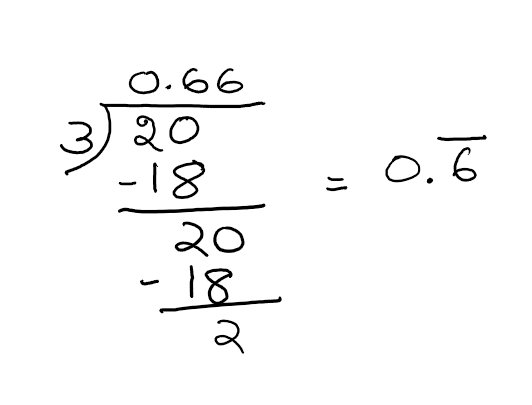$(ii)$ In this problem, the remainder $2$ repeats after the second step, making the expansion $1.8\overline{3}$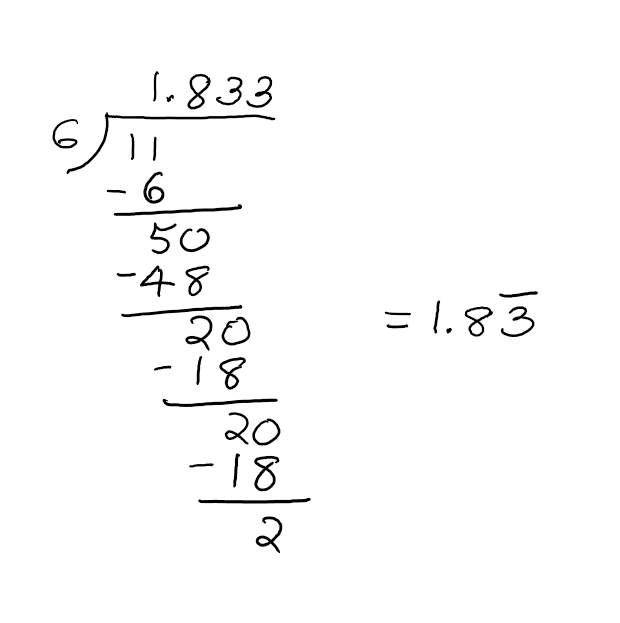$(iii)$ In this problem, $5$ is the repeating remainder. Thus we have $\frac{5}{9} = 0.\overline{5}$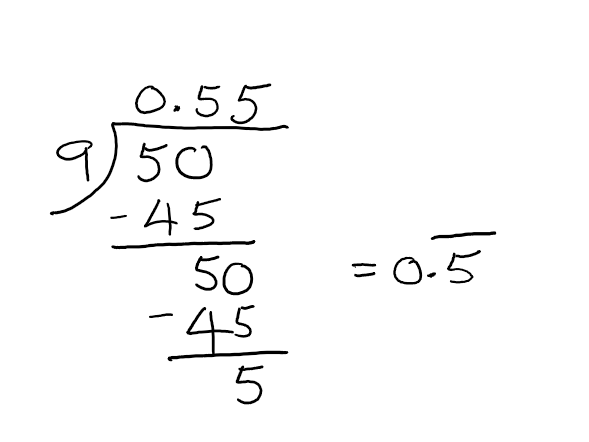$(iv)$ Here, we have remainders $9$ and $2$ repeating alternately. So, we have $\frac{11}{20} = 0.\overline{18}$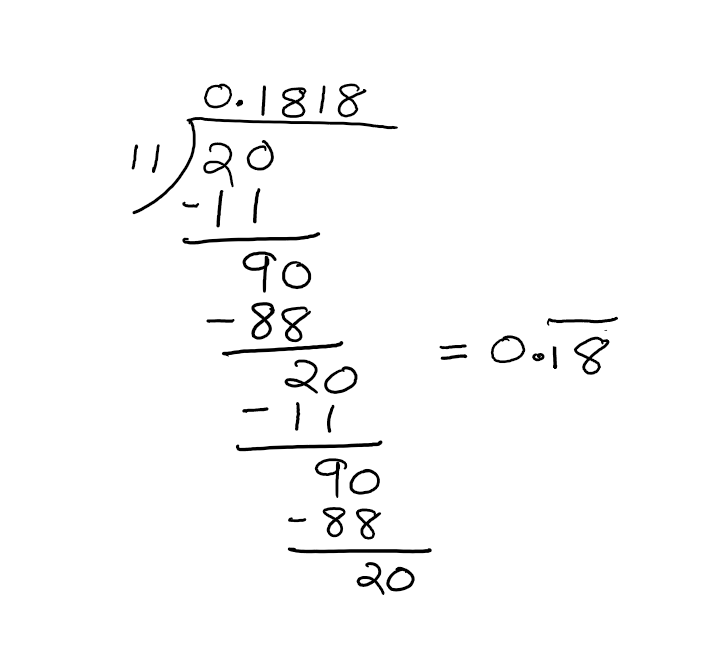Following examples illustrate process of converting recurring decimal expansions into fractions:

#### Example 4:

Express each of the following decimals as fractions: $(i) 0.\overline{6}; \quad (ii) 2.3\overline{5}; \quad (iii) 1.\overline{36}$.

Solution:

$(i)$ let $x = 0.666666.........$. Then, \begin{eqnarray} 10x &=& 6.666666......\\(-) x &=& 0.6666666......\\ 9x &=& 6\\ x &=& \frac{6}{9}\\ &=& \frac{2}{3}\\ \end{eqnarray}
$(ii)$ Let $x = 2.3\overline{5}$. Then, \begin{eqnarray} 100x &=& 235.5555555......\\ (-)10x &=& 23.5555555........\\ 90x &=& 212\\ x &=& \frac{212}{90}\\ &=& \frac{106}{90}\\ &=& 2\frac{16}{45}\\ \end{eqnarray}
$(iii)$ Let $x = 1.\overline{36}$. Then, \begin{eqnarray} 100x &=& 136.363636363.......\\ (-) x &=& 1.363636363.......\\ 99x &=& 135\\ x &=& \frac{135}{99}\\ &=& \frac{15}{11}\\ &=& 1\frac{4}{11}\\ \end{eqnarray}

In conclusion, we infer that the decimal expansion of a rational number is either terminating or repeating (recurring).

## Practice Problems

For problems #1 - 5, without actual division, determine whether each rational number has terminating or repeating expansion.

Terminating Decimal.

Terminating Decimal.

Repeating Decimal.

Repeating Decimal.

Terminating Decimal.

For problems #6 - 10, determine whether each fraction has terminating or recurring decimal expansion. Also, write their decimal expansion.

Terminating decimal. $2.3$

Terminating Decimal. $2.0425$

Recurring Decimal. $0.\overline{380952}$

Recurring Decimal. $0.\overline{45}$

Recurring Decimal. $0.6\overline{4}$

For each of problems $11 - 16$, determine whether the given decimal expansion is a rational or irrational number. If it is a rational number, then find the fraction form of it.

Rational number. $\frac{21}{50}$

Rational number. $5\frac{1}{3}$

Irrational number.

Rational number. $6\frac{11}{45}$;

Irrational number.

Rational number. $1\frac{7}{30}$